Appendix 5: Calculation of the thermodynamic state of a real fluid with a Bender equation of state
released 7.12.99, updated 9.12.99

Nomenclature:

latin symbols:

CP0(T)    ideal heat capacity (material parameter)
CV          specific heat capacity at constant volume
CP          specific heat capacity at constant pressure
ei            calculated constants of Bender equation of state
f              total fugacity
fi             partial fugacity of a component
g1,j          fixed constants of Bender equation of state
G            free enthalpy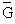molar specific free enthalpy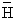molar specific enthalpy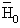reference enthalpy at reference temperature T0 and reference pressure P0
IM           defined as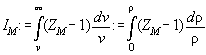kij            fit parameter for mixture rule
Mix1       fit parameter for mixture rule for ternary and higher component mixture
Mix2       fit parameter for mixture rule for ternary and higher component mixture
P0           reference pressure
P             pressure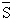molar specific entropy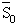reference entropy at reference temperature T0 and reference pressure P0
T             temperature
T0           reference temperature
Tc           critical temperature
v             specific molar volume
vc            critical specific molar volume
V            volume
W           work
w            specific work
x             molar ratio
ZM         real gas factor defined as Pv/RT

greek symbols: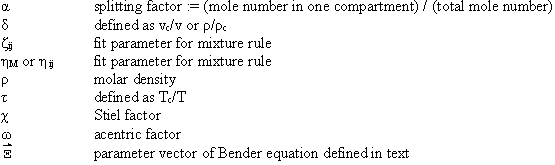indices:

upper indices:             f liquid (fluessig), g gaseous
lower indices:             i,j,k,l as component index or summation index written explicitly as number,
for example: x1 for component 1, M for mixture
numbers in brackets:  for example v(1), x(2) means at point 2
one marked number: superheated state, i.e. v(3´) or x1(2´)
double marked number: two phase state, i.e. x(2") or P(3")

How to read the formula collection
Table A1 summarizes all standard formulas from literature describing the differents aspects of a thermodynamic state. They can be found in [A1,A2,A3,A4].
In Table A2.1 the set from Table A1 is written dependent from the reduced quantities of volume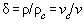and temperature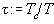To make calculation more easy it is convenient to write the formalas down in terms of ZM and IM defined as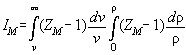.

Derivations of Table A2.1 are found in Table A2.2. They are necessary to calculate the Jacobimatrix of the Newton Raphson iteration. All formulas of the thermodynamic state are analytically calculated. Only the the derivations of ln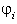with respect to xi are calculated numerically.
In Table A3.1 the Bender equation is specified, Table A3.2 contains derivations therefrom necessary for the calculation of the Jacobimatrix.
Table A4.1 specifies the mixture rules of Tsai and Shuy[A5]. Table A4.2 and A4.3 gives derivations therefrom.
For easy calculation of lnit is helpful to consider it to be dependent from (x1, x2, xi-1,xi+1,....xn)
The calculation is presented here top - down, i.e. from general to special. Programming has to be done in the reverse direction

Tab.A1:

 The thermodynamic state in standard notations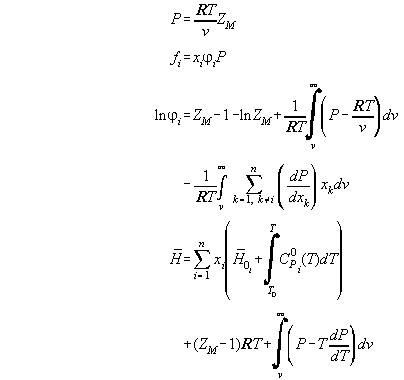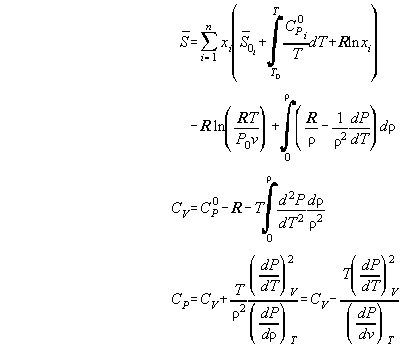Table A2.1: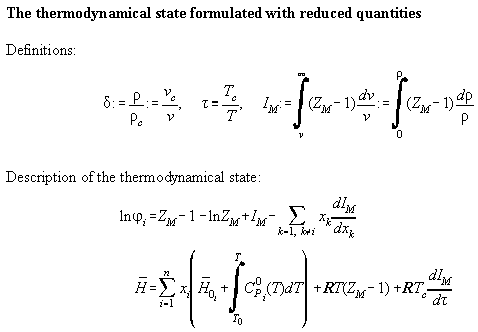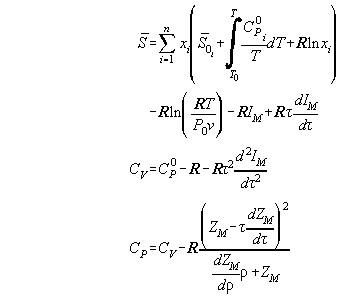Table A2.2:

 Derivatives in reduced quantities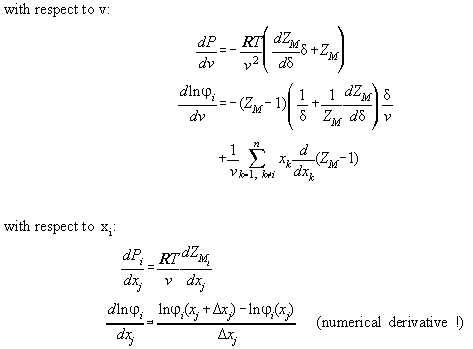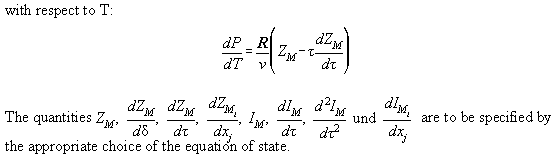Table A3.1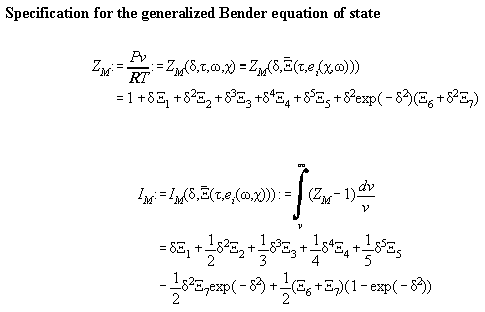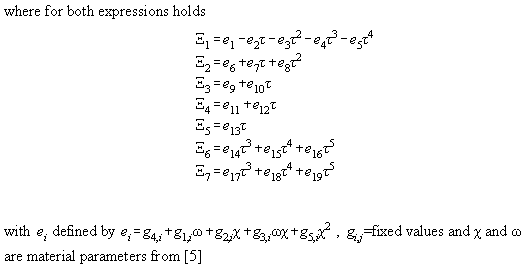Table A3.2: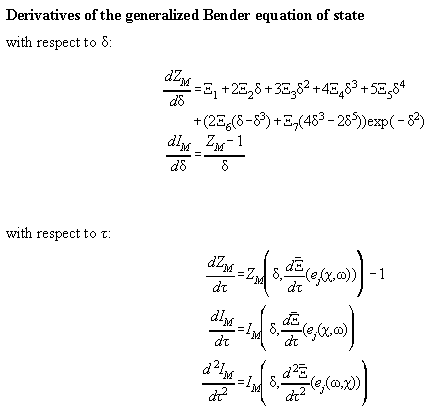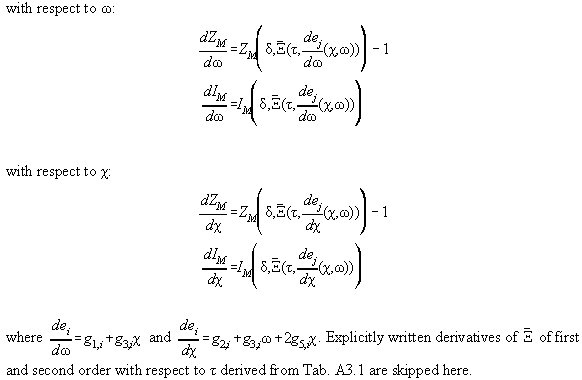Table A4.1: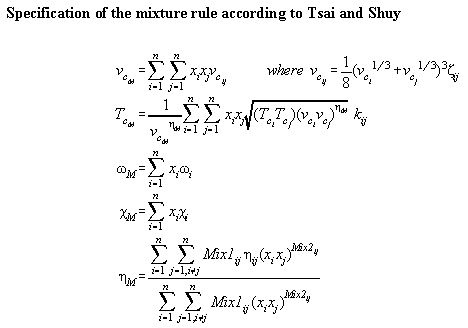Table A4.2: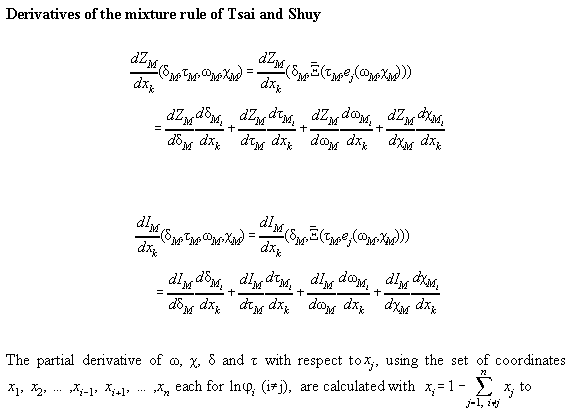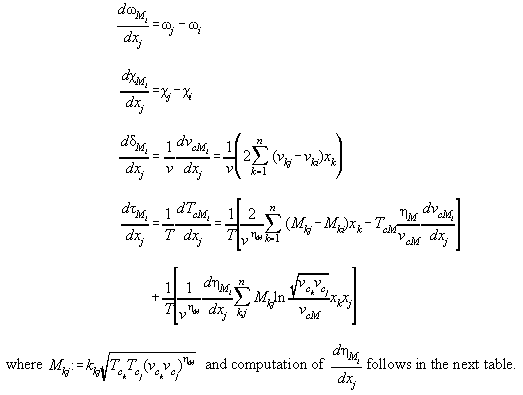Table A4.3: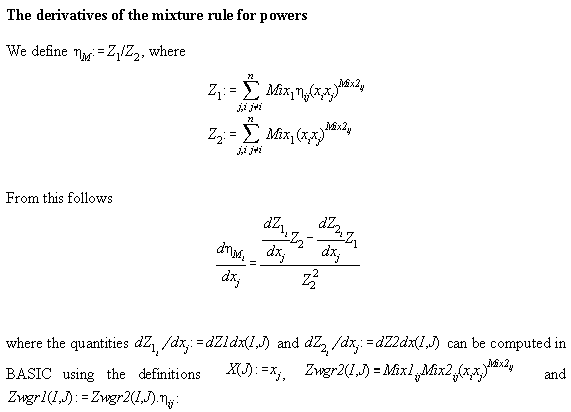FOR I=1 TO N           FOR J=1 TO N             dZ1dx(I,J)=0 : dZ2dx(I,J)=0           NEXT J          NEXT I          FOR I=1 TO N           FOR J=I+1 TO N            FOR K=1 TO N             FOR L=K+1 TO N              IF I=K OR I=L THEN               dZ1dx(I,J)=dZ1dx(I,J)-Zwgr1(K,L)/X(I)               dZ2dx(I,J)=dZ2dx(I,J)-Zwgr2(K,L)/X(I)              ENDIF              IF J=L OR J=K THEN               dZ1dx(I,J)=dZ1dx(I,J)+Zwgr1(K,L)/X(J)               dZ2dx(I,J)=dZ2dx(I,J)+Zwgr2(K,L)/X(J)              ENDIF             NEXT L            NEXT K            dZ1dx(J,I)=-dZ1dx(I,J) : dZ2dx(J,I)=-dZ2dx(I,J)           NEXT J          NEXT I

Bibliography:

[A1] Bender E., Die Berechnung von Phasengleichgewichten mit der thermischen Zustandsgleichung - dargestellt an den reinen Fluiden Argon, Stickstoff, Sauerstoff und ihren Gemischen , Habilitationsschrift, Bochum 1971

[A2] Platzer B., Die Generalisierung der Zustandsgleichung von Bender zur Berchnung von Stoffeigenschaften polarer und unpolarer Fluide und derer Gemische, Dissertation, Fachbereich Maschinenbau, Uni Kaiserslautern 1990

[A3] Stephan K., Mayinger F. Thermodynamik, Springer Verlag, New York 1988

[A4] Polt A., Zur Beschreibung von reinen Fluiden mit BWR-Gleichungen, Fachbereich Maschinenbau, Uni Kaiserslautern 1986/87

[A5] Tsai F., Shuy J.J., Prediction of high pressure vapor-liquid equilibria by the corresponding-states principle, J. Chin.Chem.Engn. 16 (1985) 157

>>>>>>>>>>>>>>>>>>>>>>>>>>>>>>   back to the main document